Скачать презентацию Capacity Limits of Wireless Channels with Multiple Antennas

c42df6b7abba7ed2eab5a2b69086a006.ppt

• Количество слайдов: 54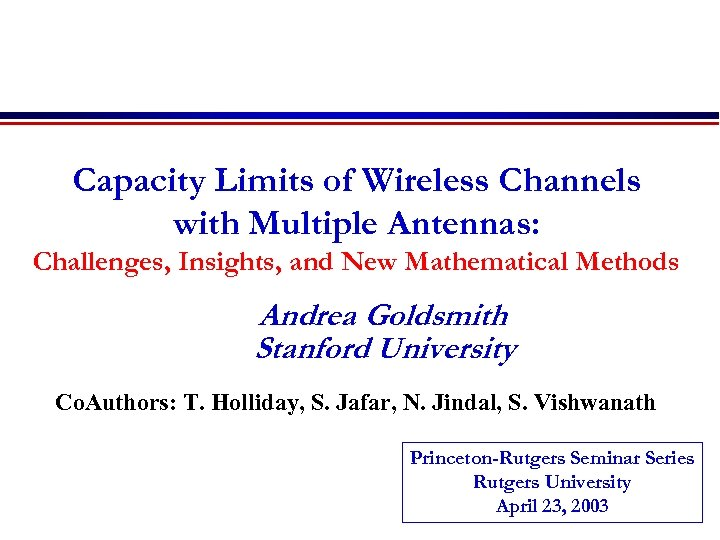Capacity Limits of Wireless Channels with Multiple Antennas: Challenges, Insights, and New Mathematical Methods Andrea Goldsmith Stanford University Co. Authors: T. Holliday, S. Jafar, N. Jindal, S. Vishwanath Princeton-Rutgers Seminar Series Rutgers University April 23, 2003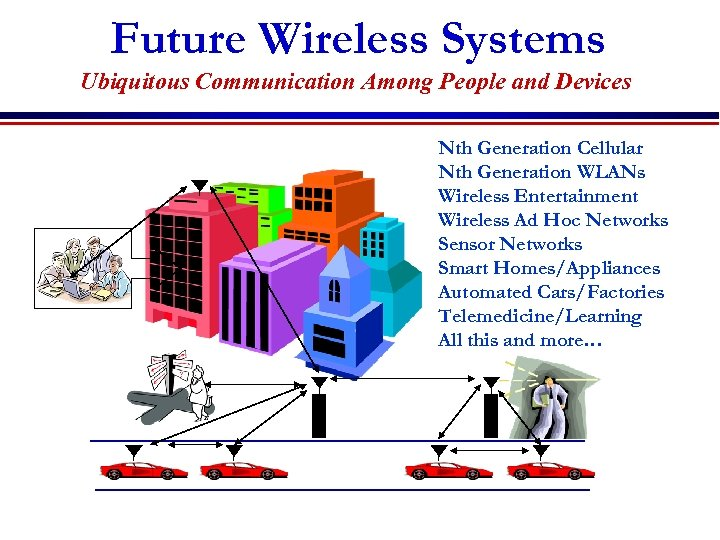Future Wireless Systems Ubiquitous Communication Among People and Devices Nth Generation Cellular Nth Generation WLANs Wireless Entertainment Wireless Ad Hoc Networks Sensor Networks Smart Homes/Appliances Automated Cars/Factories Telemedicine/Learning All this and more…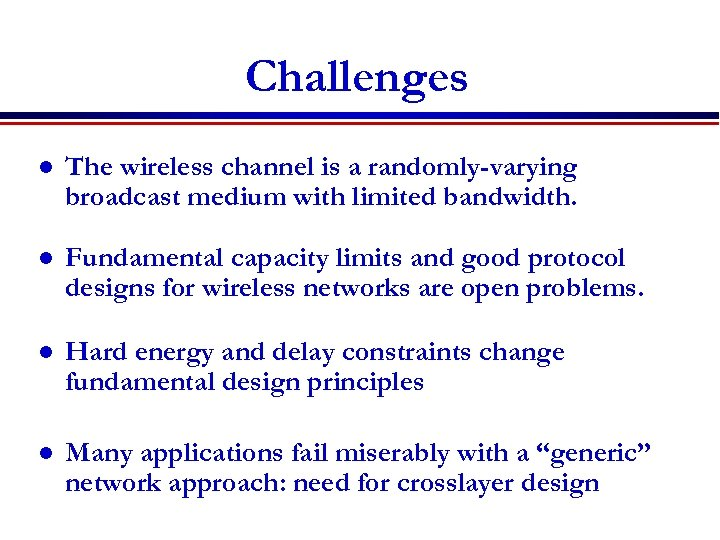Challenges l The wireless channel is a randomly-varying broadcast medium with limited bandwidth. l Fundamental capacity limits and good protocol designs for wireless networks are open problems. l Hard energy and delay constraints change fundamental design principles l Many applications fail miserably with a “generic” network approach: need for crosslayer design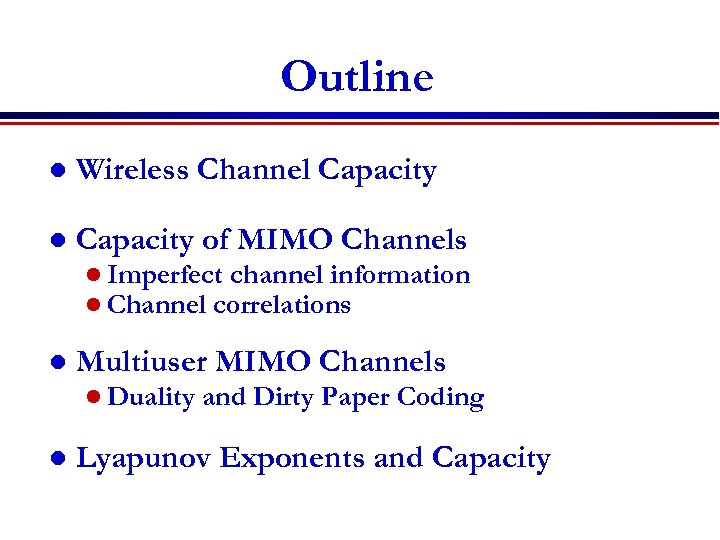Outline l Wireless Channel Capacity of MIMO Channels l Imperfect channel information l Channel correlations l Multiuser MIMO Channels l Duality l and Dirty Paper Coding Lyapunov Exponents and Capacity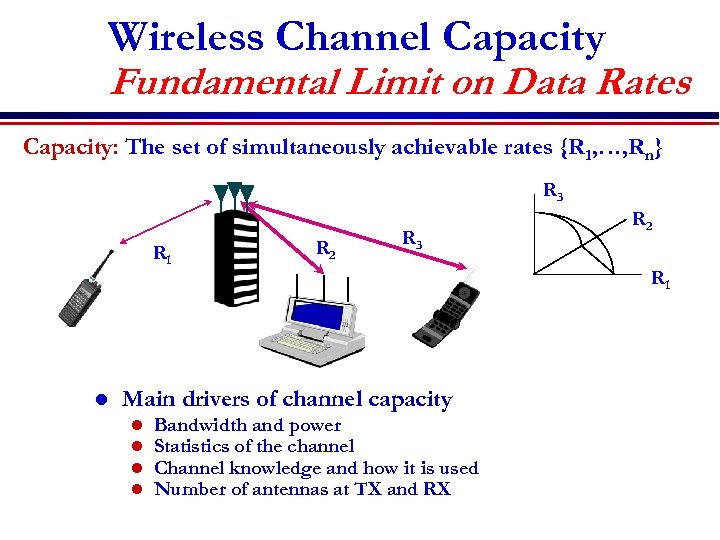Wireless Channel Capacity Fundamental Limit on Data Rates Capacity: The set of simultaneously achievable rates {R 1, …, Rn} R 3 R 1 l R 2 R 3 Main drivers of channel capacity l l Bandwidth and power Statistics of the channel Channel knowledge and how it is used Number of antennas at TX and RX R 2 R 1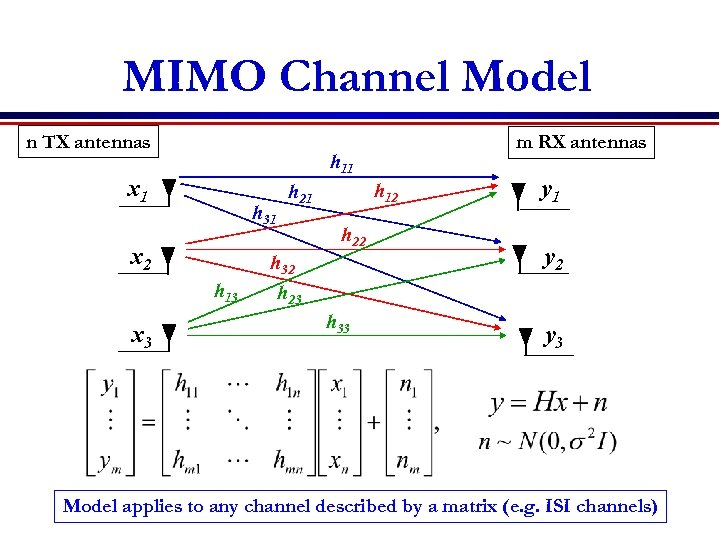MIMO Channel Model n TX antennas h 11 x 1 h 31 x 2 h 12 h 21 h 22 h 32 h 13 x 3 m RX antennas y 1 y 2 h 23 h 33 y 3 Model applies to any channel described by a matrix (e. g. ISI channels)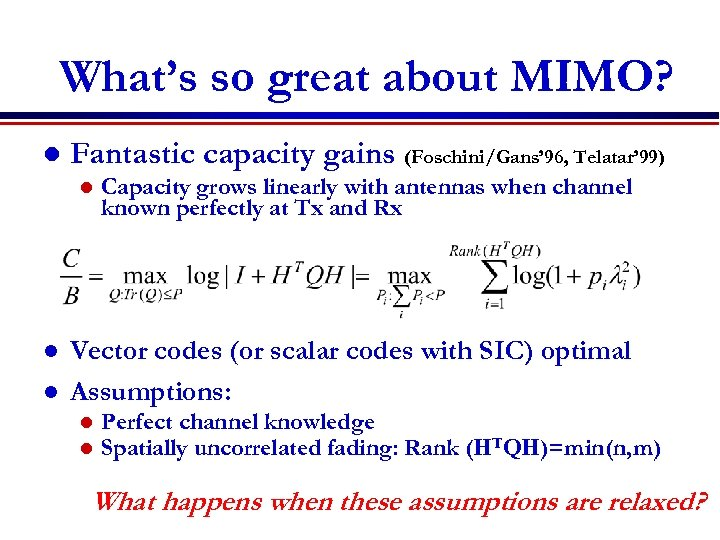What’s so great about MIMO? l Fantastic capacity gains (Foschini/Gans’ 96, Telatar’ 99) l l l Capacity grows linearly with antennas when channel known perfectly at Tx and Rx Vector codes (or scalar codes with SIC) optimal Assumptions: l l Perfect channel knowledge Spatially uncorrelated fading: Rank (HTQH)=min(n, m) What happens when these assumptions are relaxed?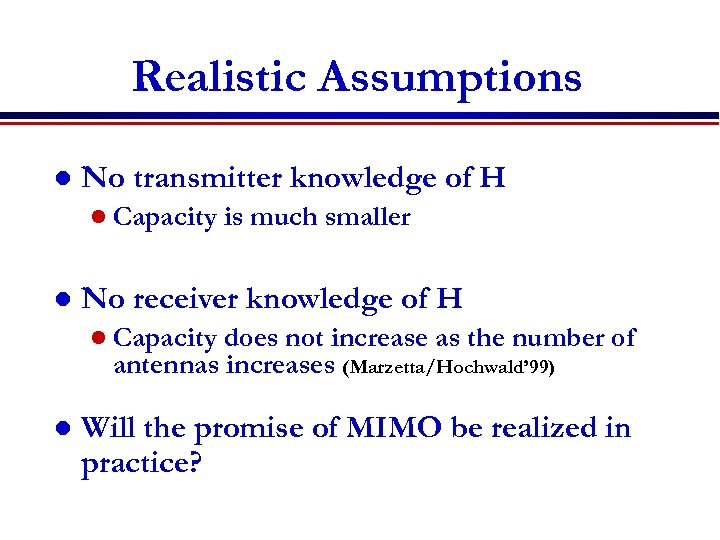Realistic Assumptions l No transmitter knowledge of H l Capacity l is much smaller No receiver knowledge of H l Capacity does not increase as the number of antennas increases (Marzetta/Hochwald’ 99) l Will the promise of MIMO be realized in practice?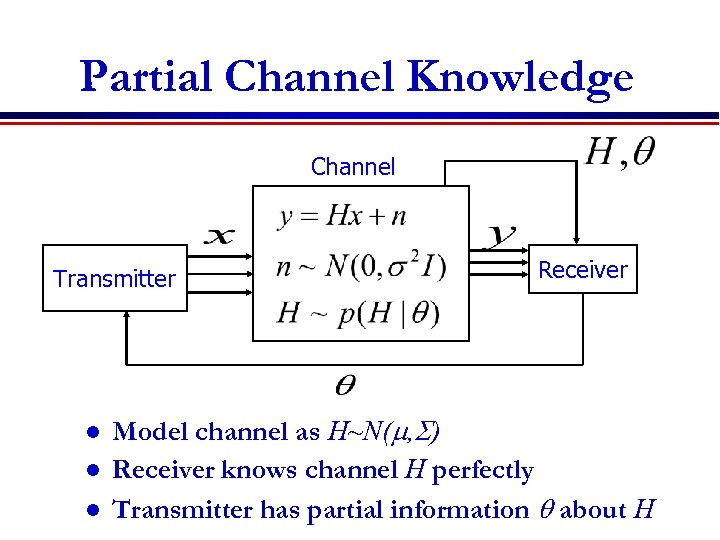Partial Channel Knowledge Channel Transmitter l l l Receiver Model channel as H~N(m, S) Receiver knows channel H perfectly Transmitter has partial information q about H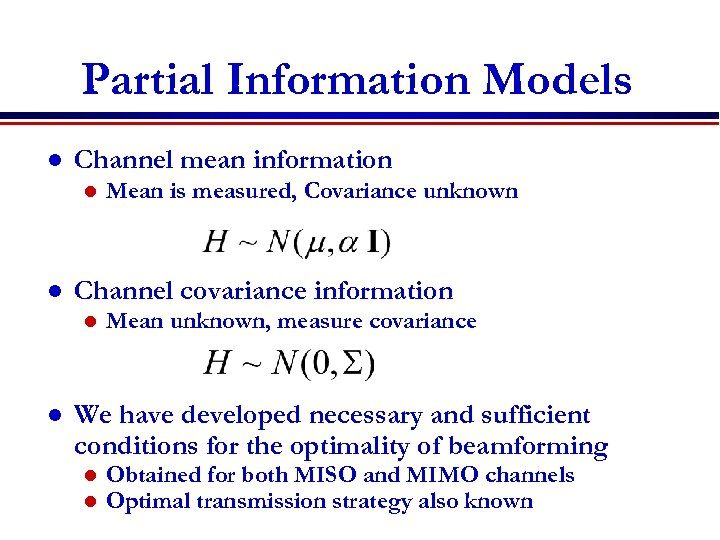Partial Information Models l Channel mean information l l Channel covariance information l l Mean is measured, Covariance unknown Mean unknown, measure covariance We have developed necessary and sufficient conditions for the optimality of beamforming l l Obtained for both MISO and MIMO channels Optimal transmission strategy also known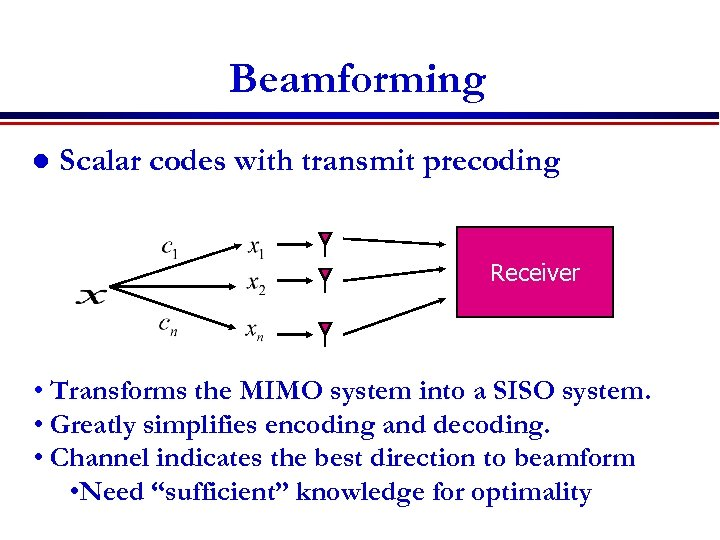Beamforming l Scalar codes with transmit precoding Receiver • Transforms the MIMO system into a SISO system. • Greatly simplifies encoding and decoding. • Channel indicates the best direction to beamform • Need “sufficient” knowledge for optimality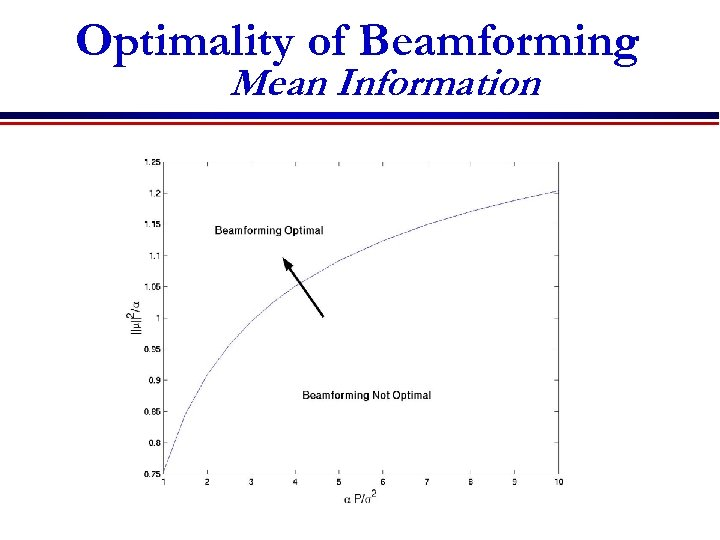Optimality of Beamforming Mean Information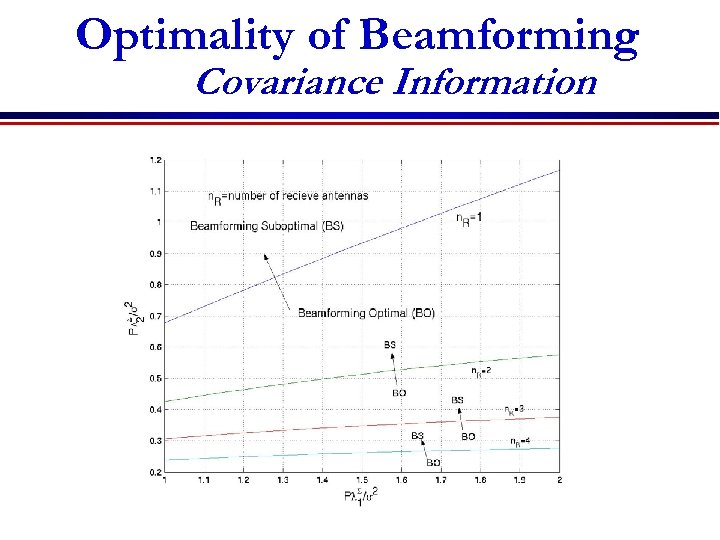Optimality of Beamforming Covariance Information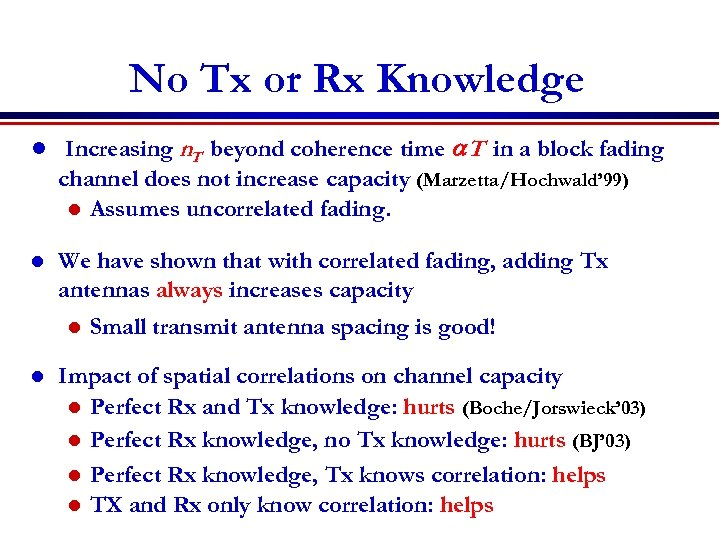No Tx or Rx Knowledge l Increasing n. T beyond coherence time a. T in a block fading channel does not increase capacity (Marzetta/Hochwald’ 99) l Assumes uncorrelated fading. l We have shown that with correlated fading, adding Tx antennas always increases capacity l l Small transmit antenna spacing is good! Impact of spatial correlations on channel capacity l Perfect Rx and Tx knowledge: hurts (Boche/Jorswieck’ 03) l Perfect Rx knowledge, no Tx knowledge: hurts (BJ’ 03) l Perfect Rx knowledge, Tx knows correlation: helps l TX and Rx only know correlation: helps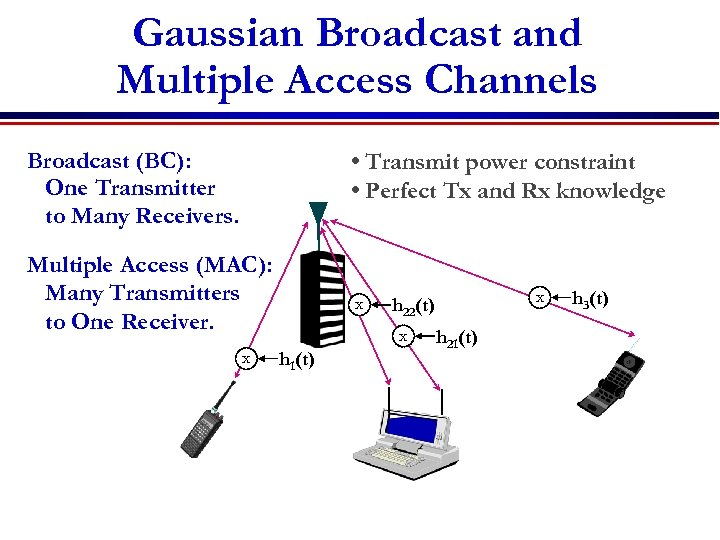Gaussian Broadcast and Multiple Access Channels Broadcast (BC): One Transmitter to Many Receivers. • Transmit power constraint • Perfect Tx and Rx knowledge Multiple Access (MAC): Many Transmitters to One Receiver. x x x h 1(t) x h 22(t) h 21(t) h 3(t)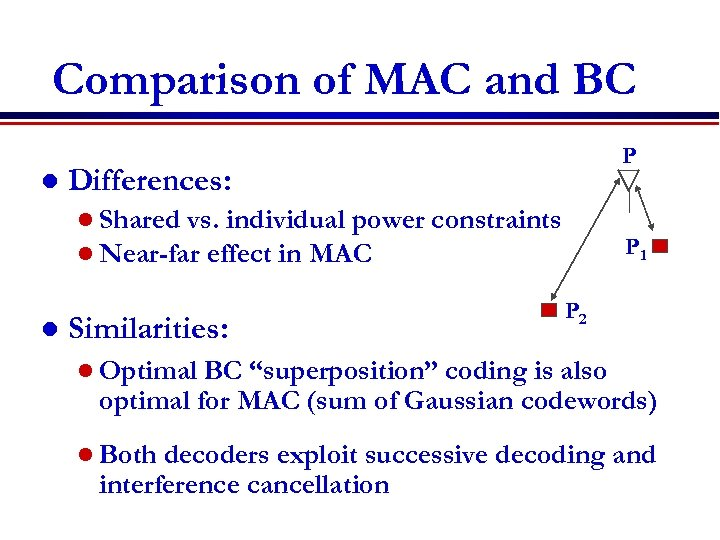Comparison of MAC and BC l Differences: l Shared vs. individual power l Near-far effect in MAC l P Similarities: constraints P 1 P 2 l Optimal BC “superposition” coding is also optimal for MAC (sum of Gaussian codewords) l Both decoders exploit successive decoding and interference cancellation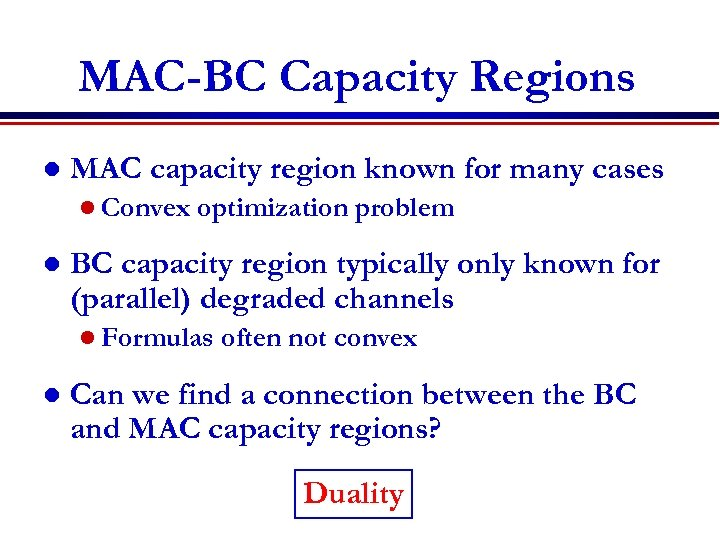MAC-BC Capacity Regions l MAC capacity region known for many cases l Convex l optimization problem BC capacity region typically only known for (parallel) degraded channels l Formulas l often not convex Can we find a connection between the BC and MAC capacity regions? Duality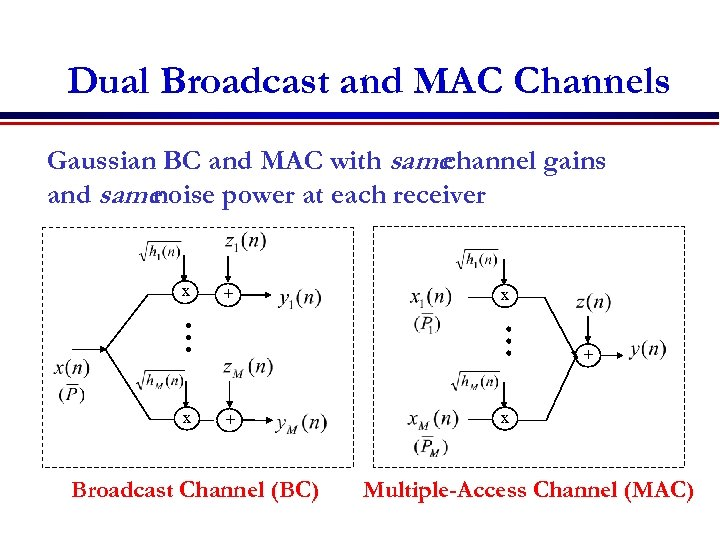Dual Broadcast and MAC Channels Gaussian BC and MAC with same channel gains and same noise power at each receiver x + x + Broadcast Channel (BC) x Multiple-Access Channel (MAC)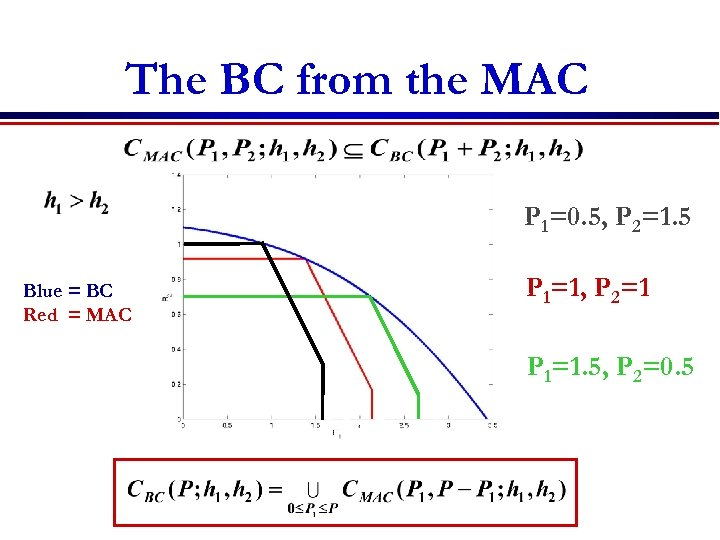The BC from the MAC P 1=0. 5, P 2=1. 5 Blue = BC Red = MAC P 1=1, P 2=1 P 1=1. 5, P 2=0. 5 MAC with sum-power constraint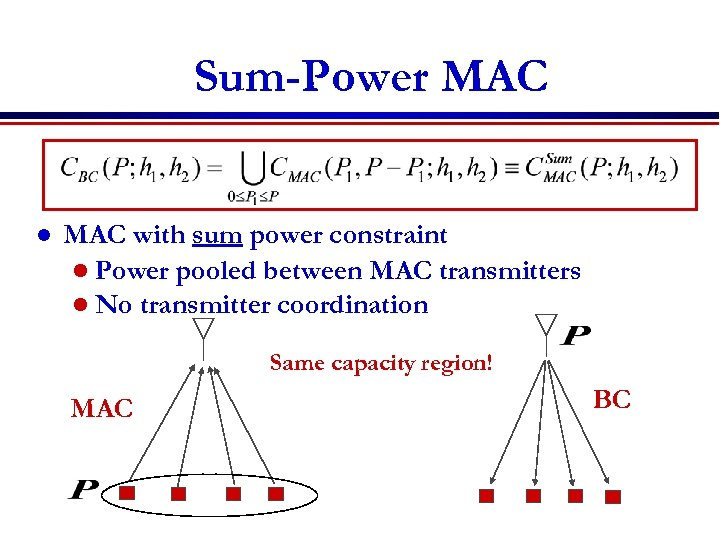Sum-Power MAC l MAC with sum power constraint l Power pooled between MAC transmitters l No transmitter coordination Same capacity region! MAC BC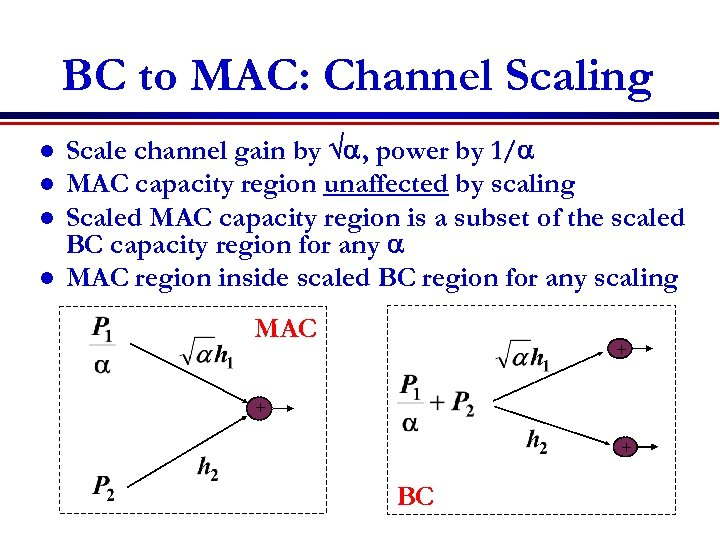BC to MAC: Channel Scaling l l Scale channel gain by a, power by 1/a MAC capacity region unaffected by scaling Scaled MAC capacity region is a subset of the scaled BC capacity region for any a MAC region inside scaled BC region for any scaling MAC + + + BC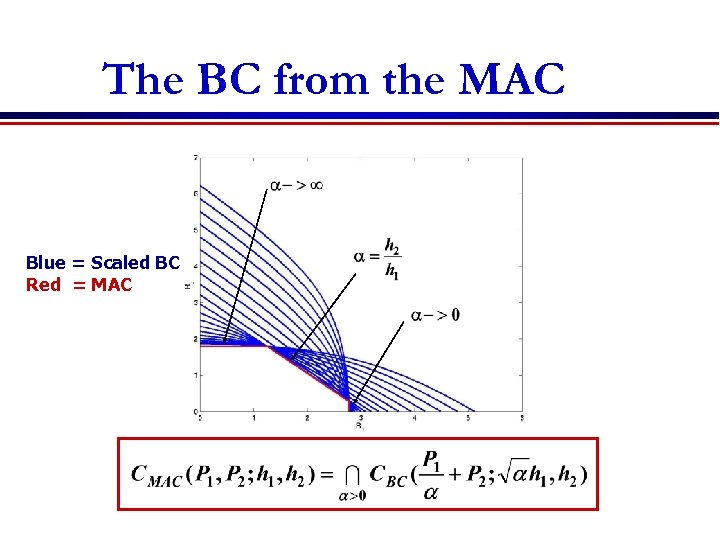The BC from the MAC Blue = Scaled BC Red = MAC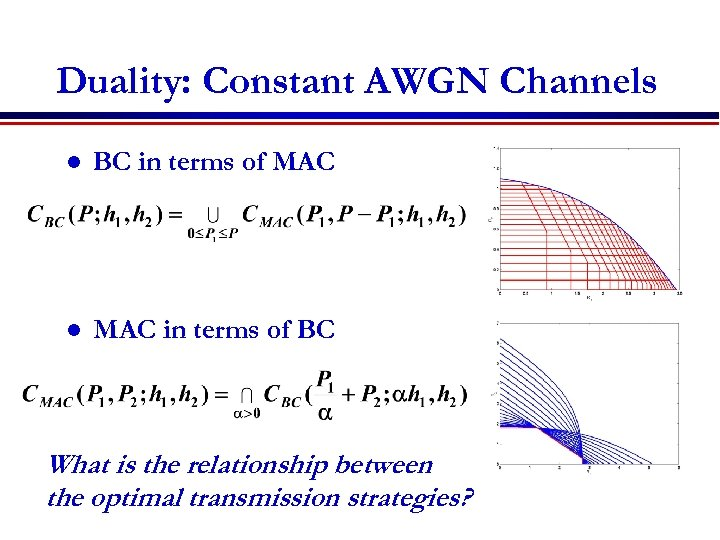Duality: Constant AWGN Channels l BC in terms of MAC l MAC in terms of BC What is the relationship between the optimal transmission strategies?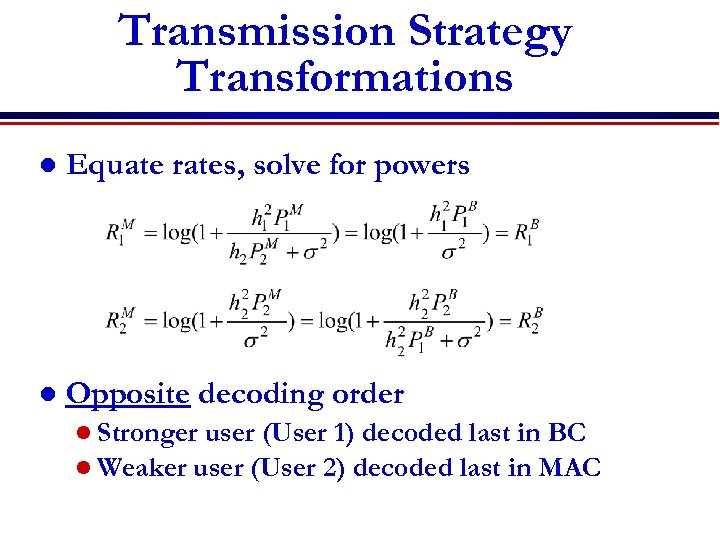Transmission Strategy Transformations l Equate rates, solve for powers l Opposite decoding order l Stronger user (User 1) decoded last in BC l Weaker user (User 2) decoded last in MAC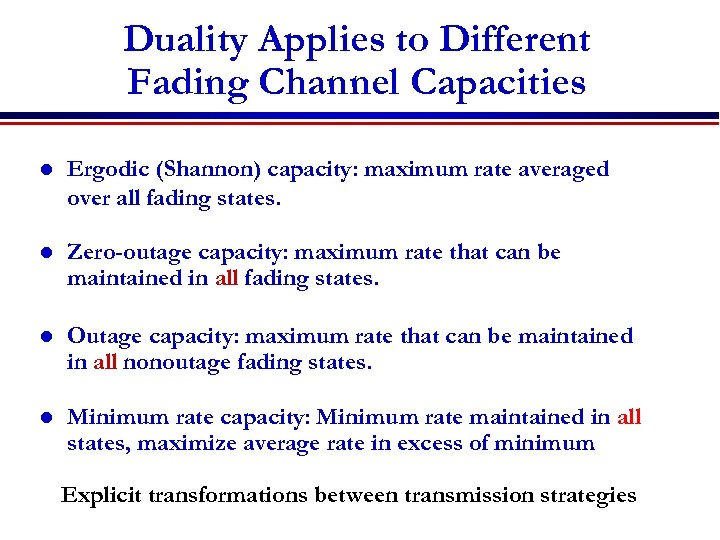Duality Applies to Different Fading Channel Capacities l Ergodic (Shannon) capacity: maximum rate averaged over all fading states. l Zero-outage capacity: maximum rate that can be maintained in all fading states. l Outage capacity: maximum rate that can be maintained in all nonoutage fading states. l Minimum rate capacity: Minimum rate maintained in all states, maximize average rate in excess of minimum Explicit transformations between transmission strategies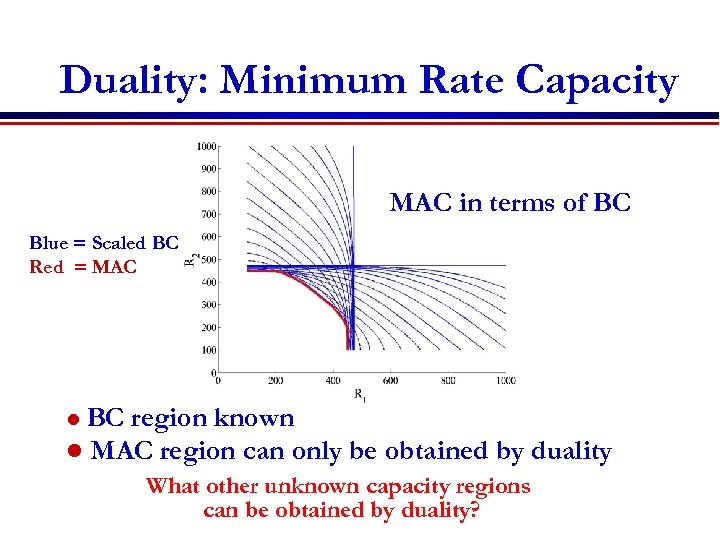Duality: Minimum Rate Capacity MAC in terms of BC Blue = Scaled BC Red = MAC BC region known l MAC region can only be obtained by duality l What other unknown capacity regions can be obtained by duality?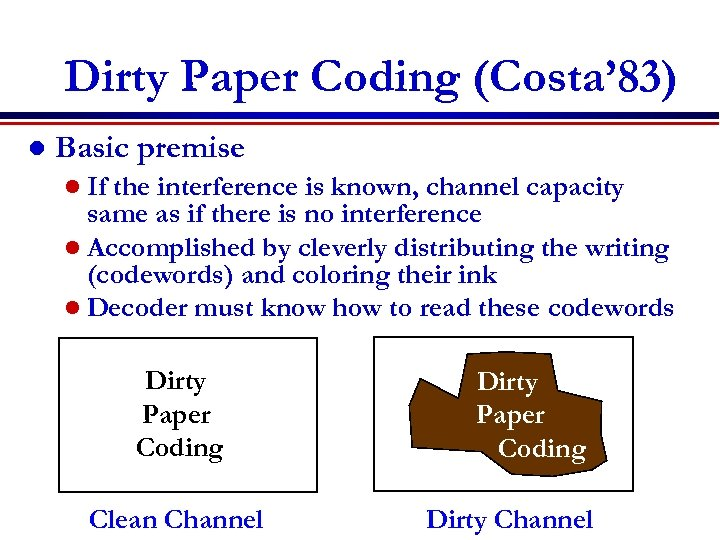Dirty Paper Coding (Costa’ 83) l Basic premise l If the interference is known, channel capacity same as if there is no interference l Accomplished by cleverly distributing the writing (codewords) and coloring their ink l Decoder must know how to read these codewords Dirty Paper Coding Clean Channel Dirty Paper Coding Dirty Channel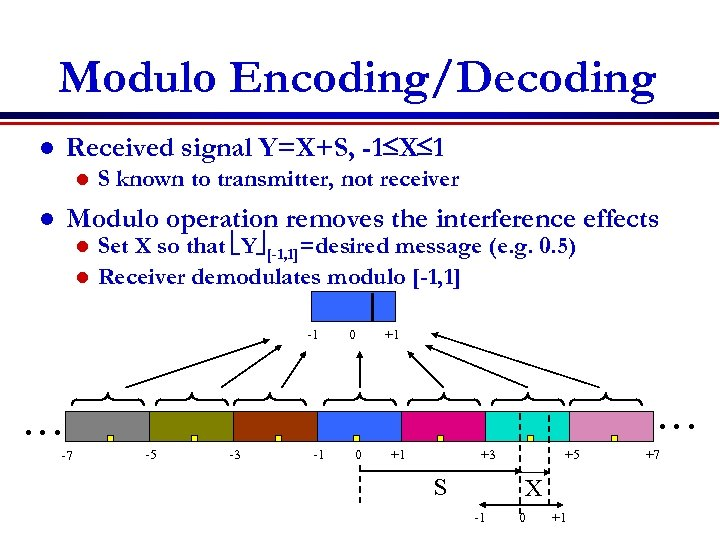Modulo Encoding/Decoding Received signal Y=X+S, -1 X 1 l l S known to transmitter, not receiver Modulo operation removes the interference effects l Set X so that Y [-1, 1]=desired message (e. g. 0. 5) l Receiver demodulates modulo [-1, 1] l -1 0 +1 … … -7 -5 -3 -1 0 +1 +3 S +5 X -1 0 +1 +7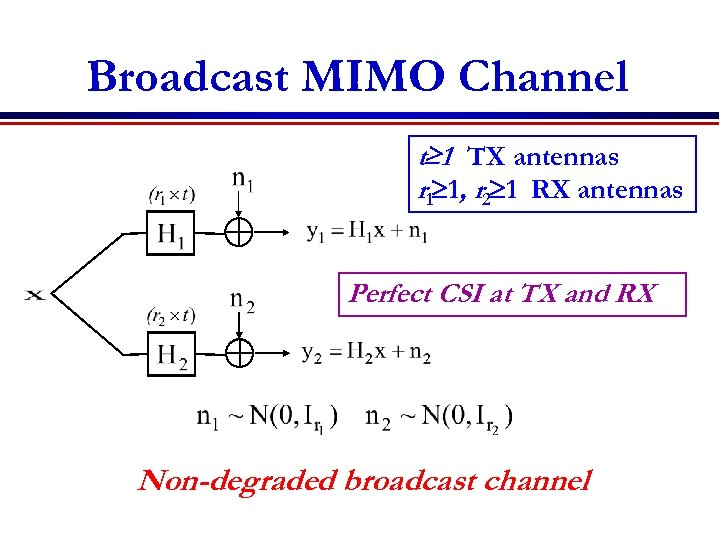Broadcast MIMO Channel t 1 TX antennas r 1 1, r 2 1 RX antennas Perfect CSI at TX and RX Non-degraded broadcast channel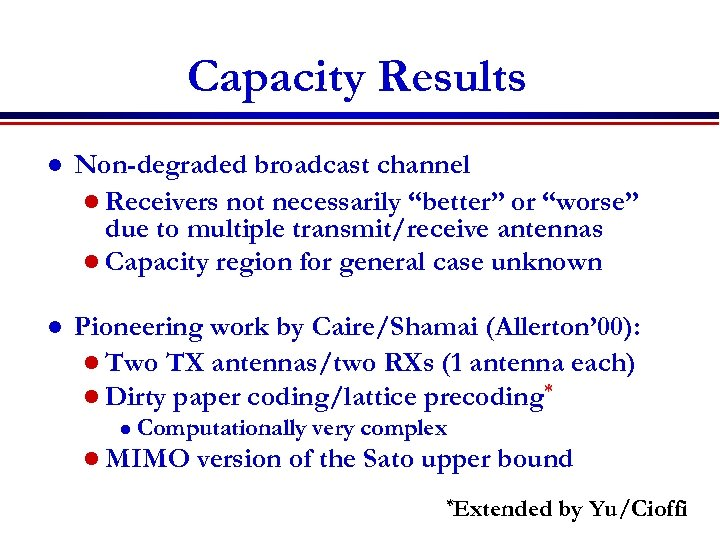Capacity Results l Non-degraded broadcast channel l Receivers not necessarily “better” or “worse” due to multiple transmit/receive antennas l Capacity region for general case unknown l Pioneering work by Caire/Shamai (Allerton’ 00): l Two TX antennas/two RXs (1 antenna each) l Dirty paper coding/lattice precoding* l Computationally very complex l MIMO version of the Sato upper bound *Extended by Yu/Cioffi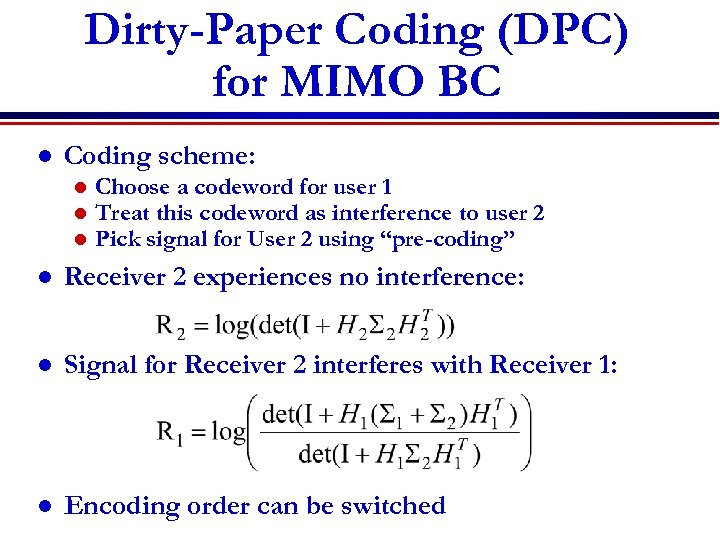Dirty-Paper Coding (DPC) for MIMO BC l Coding scheme: l l l Choose a codeword for user 1 Treat this codeword as interference to user 2 Pick signal for User 2 using “pre-coding” l Receiver 2 experiences no interference: l Signal for Receiver 2 interferes with Receiver 1: l Encoding order can be switched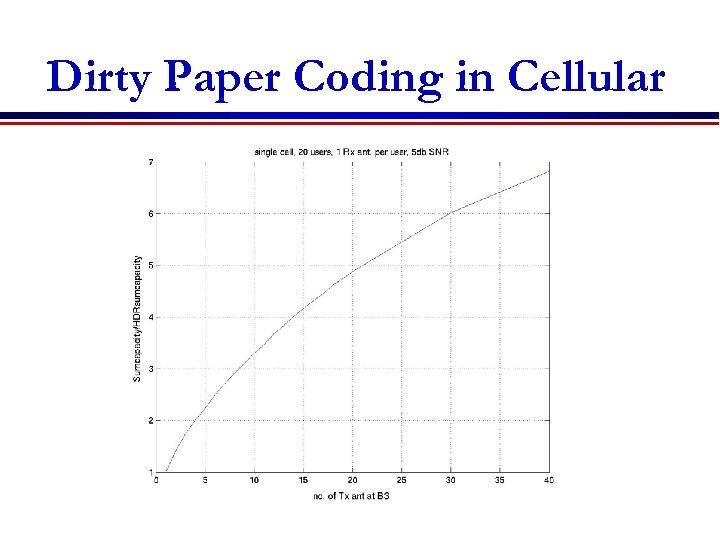Dirty Paper Coding in Cellular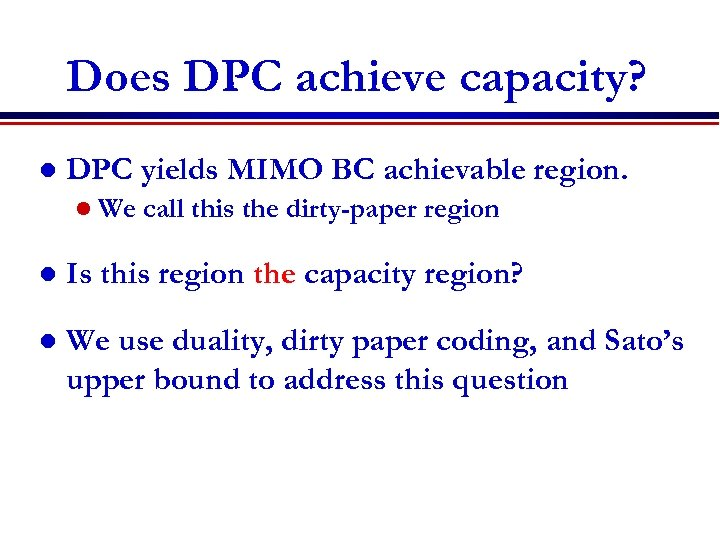Does DPC achieve capacity? l DPC yields MIMO BC achievable region. l We call this the dirty-paper region l Is this region the capacity region? l We use duality, dirty paper coding, and Sato’s upper bound to address this question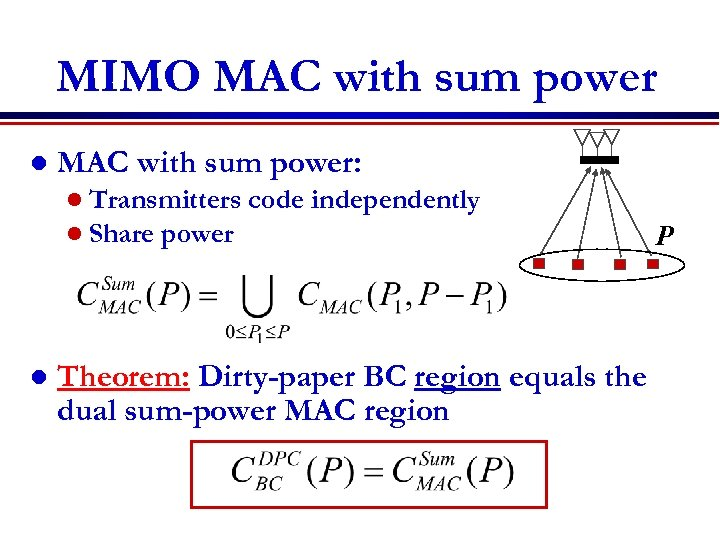MIMO MAC with sum power l MAC with sum power: l Transmitters l Share power l code independently Theorem: Dirty-paper BC region equals the dual sum-power MAC region P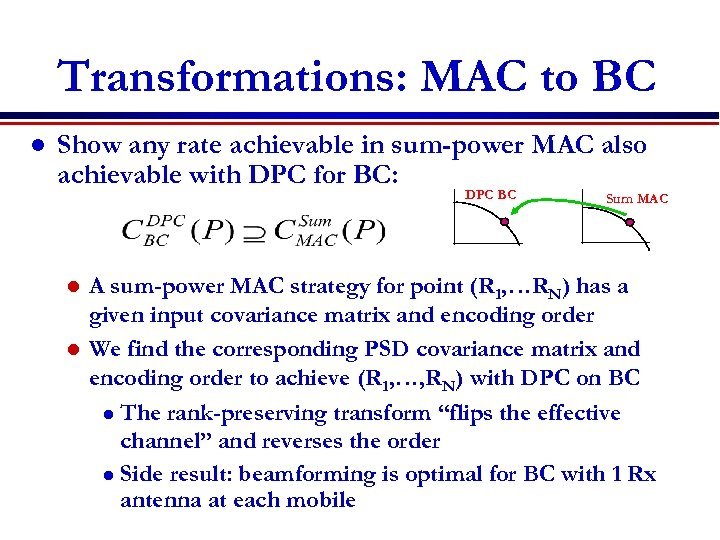Transformations: MAC to BC l Show any rate achievable in sum-power MAC also achievable with DPC for BC: DPC BC Sum MAC A sum-power MAC strategy for point (R 1, …RN) has a given input covariance matrix and encoding order l We find the corresponding PSD covariance matrix and encoding order to achieve (R 1, …, RN) with DPC on BC l The rank-preserving transform “flips the effective channel” and reverses the order l Side result: beamforming is optimal for BC with 1 Rx antenna at each mobile l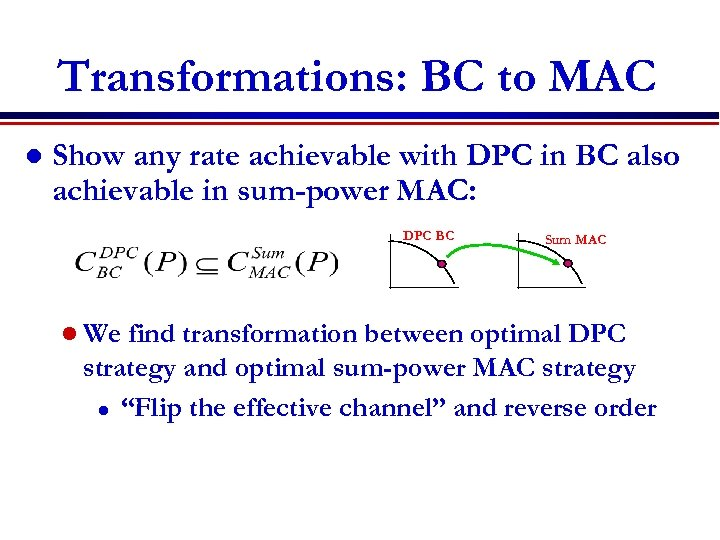Transformations: BC to MAC l Show any rate achievable with DPC in BC also achievable in sum-power MAC: DPC BC l We Sum MAC find transformation between optimal DPC strategy and optimal sum-power MAC strategy l “Flip the effective channel” and reverse order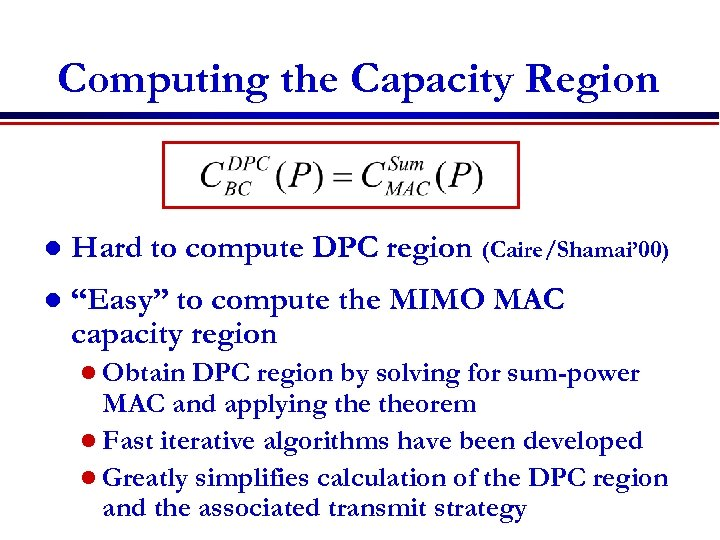Computing the Capacity Region l Hard to compute DPC region (Caire/Shamai’ 00) l “Easy” to compute the MIMO MAC capacity region l Obtain DPC region by solving for sum-power MAC and applying theorem l Fast iterative algorithms have been developed l Greatly simplifies calculation of the DPC region and the associated transmit strategy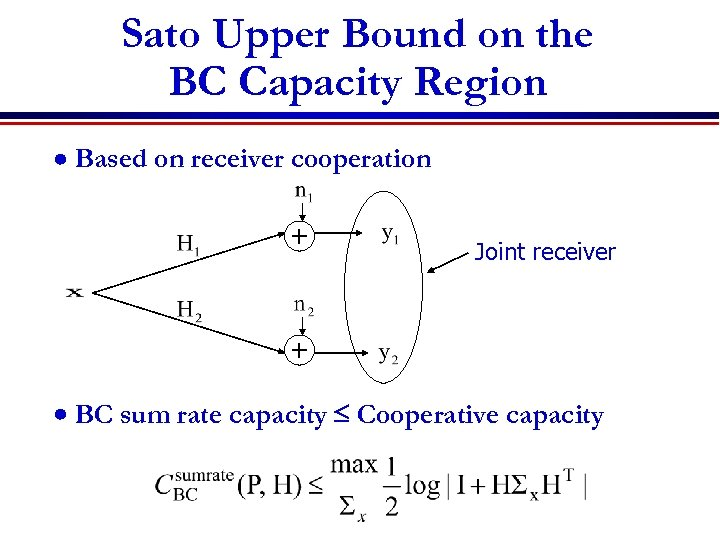Sato Upper Bound on the BC Capacity Region Based on receiver cooperation + Joint receiver + BC sum rate capacity Cooperative capacity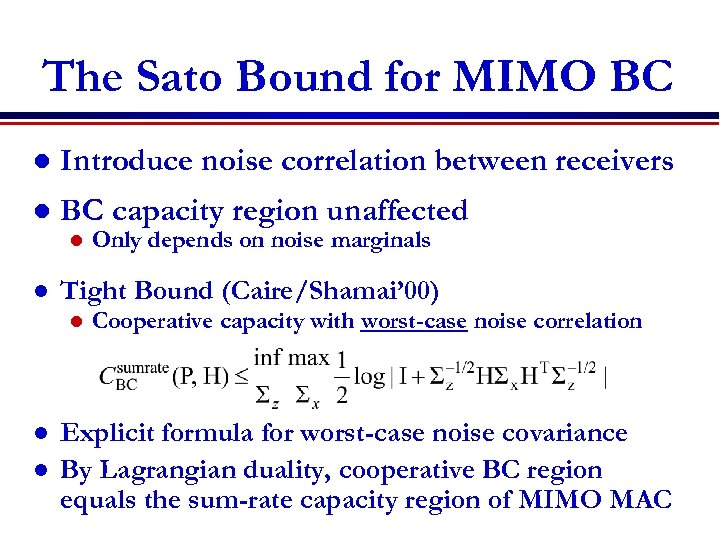The Sato Bound for MIMO BC l Introduce noise correlation between receivers l BC capacity region unaffected l l Tight Bound (Caire/Shamai’ 00) l l l Only depends on noise marginals Cooperative capacity with worst-case noise correlation Explicit formula for worst-case noise covariance By Lagrangian duality, cooperative BC region equals the sum-rate capacity region of MIMO MAC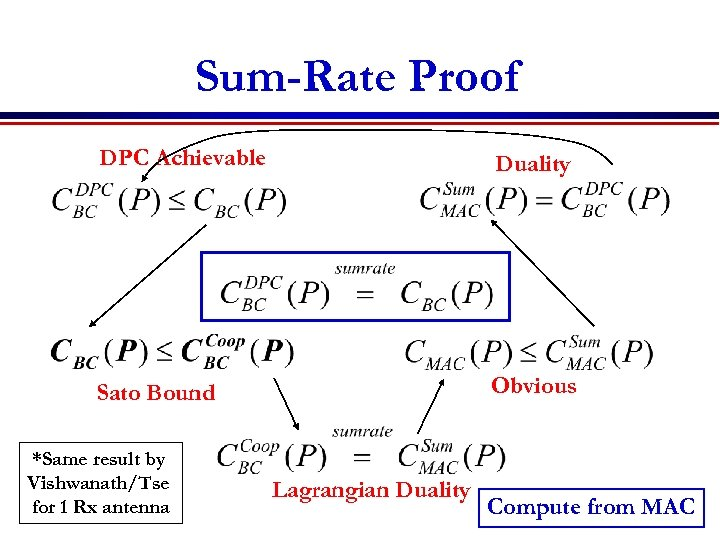Sum-Rate Proof DPC Achievable Duality Sato Bound Obvious *Same result by Vishwanath/Tse for 1 Rx antenna Lagrangian Duality Compute from MAC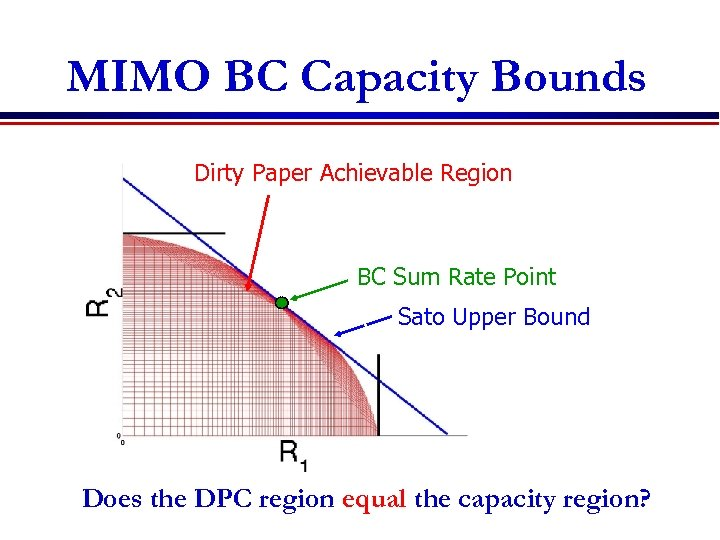MIMO BC Capacity Bounds Single User Capacity Bounds Dirty Paper Achievable Region BC Sum Rate Point Sato Upper Bound Does the DPC region equal the capacity region?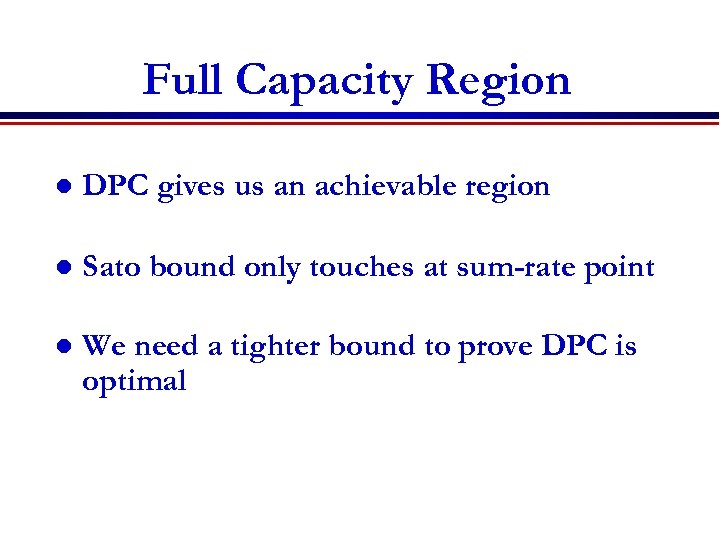Full Capacity Region l DPC gives us an achievable region l Sato bound only touches at sum-rate point l We need a tighter bound to prove DPC is optimal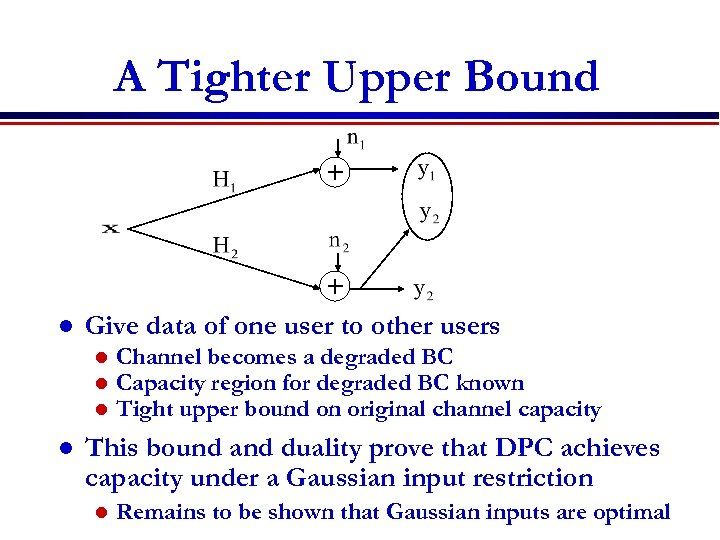A Tighter Upper Bound + l + Give data of one user to other users l l Channel becomes a degraded BC Capacity region for degraded BC known Tight upper bound on original channel capacity This bound and duality prove that DPC achieves capacity under a Gaussian input restriction l Remains to be shown that Gaussian inputs are optimal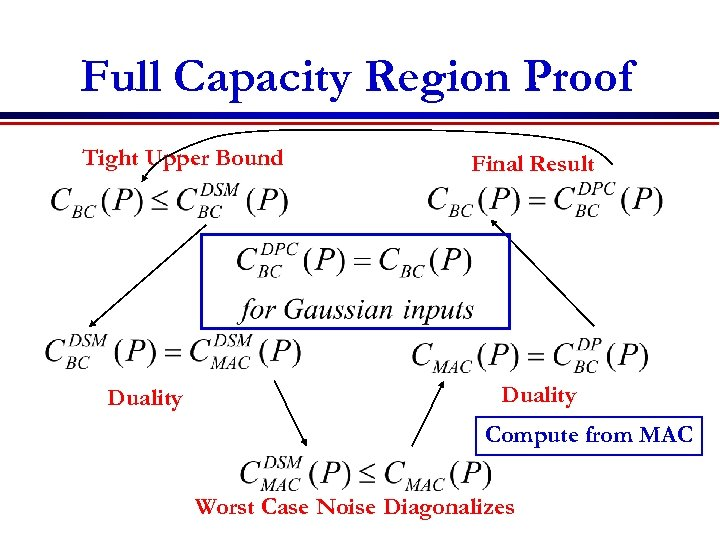Full Capacity Region Proof Tight Upper Bound Duality Final Result Duality Compute from MAC Worst Case Noise Diagonalizes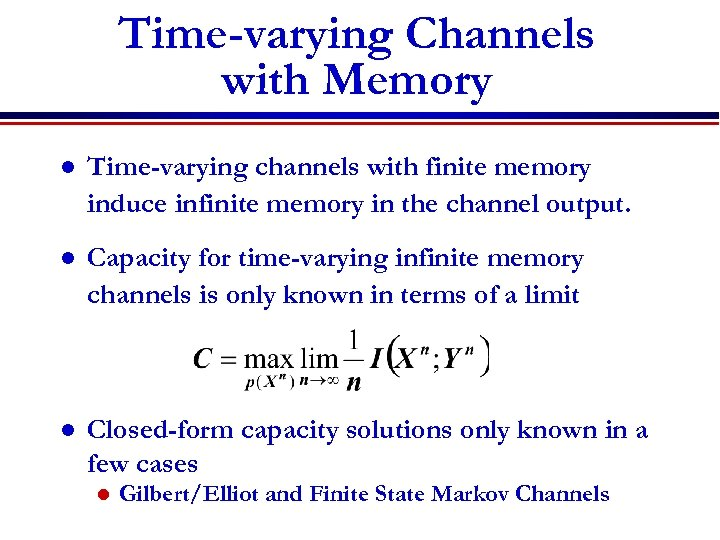Time-varying Channels with Memory l Time-varying channels with finite memory induce infinite memory in the channel output. l Capacity for time-varying infinite memory channels is only known in terms of a limit l Closed-form capacity solutions only known in a few cases l Gilbert/Elliot and Finite State Markov Channels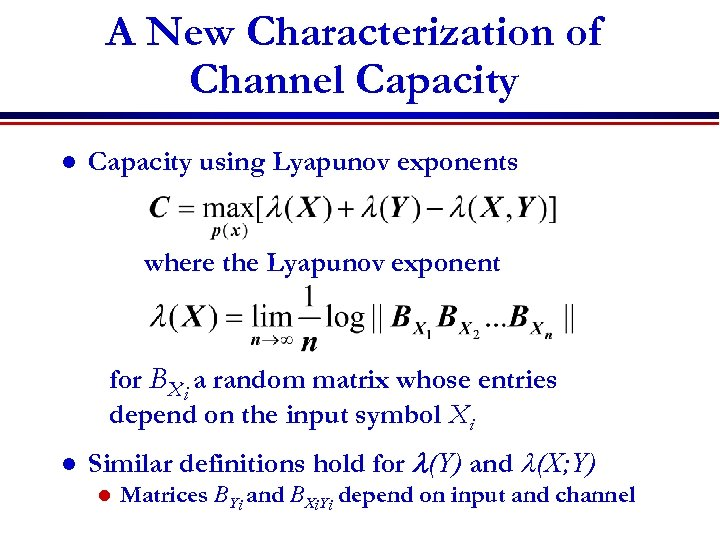A New Characterization of Channel Capacity using Lyapunov exponents where the Lyapunov exponent for BXi a random matrix whose entries depend on the input symbol Xi l Similar definitions hold for l(Y) and l(X; Y) l Matrices BYi and BXi. Yi depend on input and channel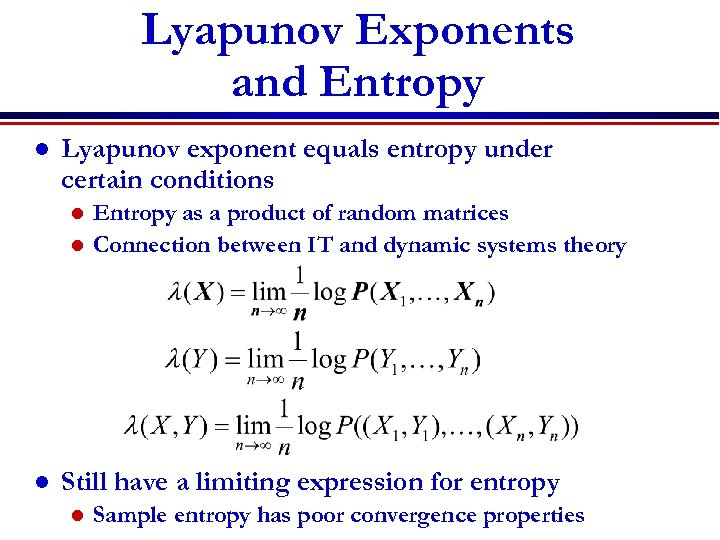Lyapunov Exponents and Entropy l Lyapunov exponent equals entropy under certain conditions Entropy as a product of random matrices l Connection between IT and dynamic systems theory l l Still have a limiting expression for entropy l Sample entropy has poor convergence properties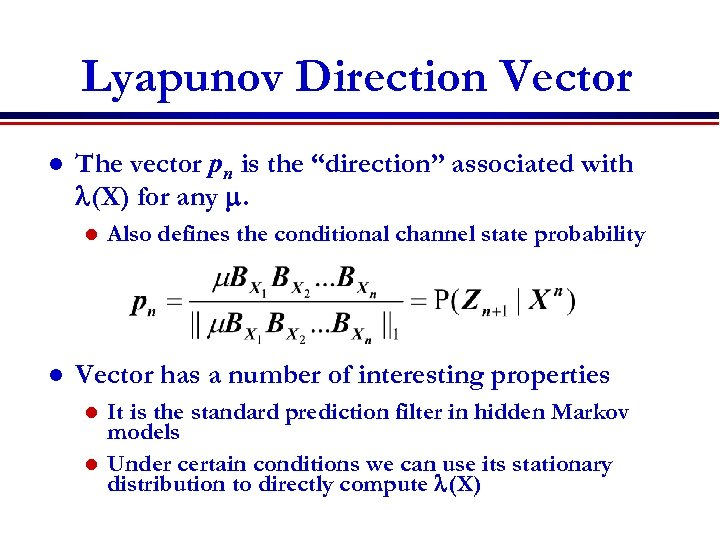Lyapunov Direction Vector l The vector pn is the “direction” associated with l(X) for any m. l l Also defines the conditional channel state probability Vector has a number of interesting properties It is the standard prediction filter in hidden Markov models l Under certain conditions we can use its stationary distribution to directly compute l(X) l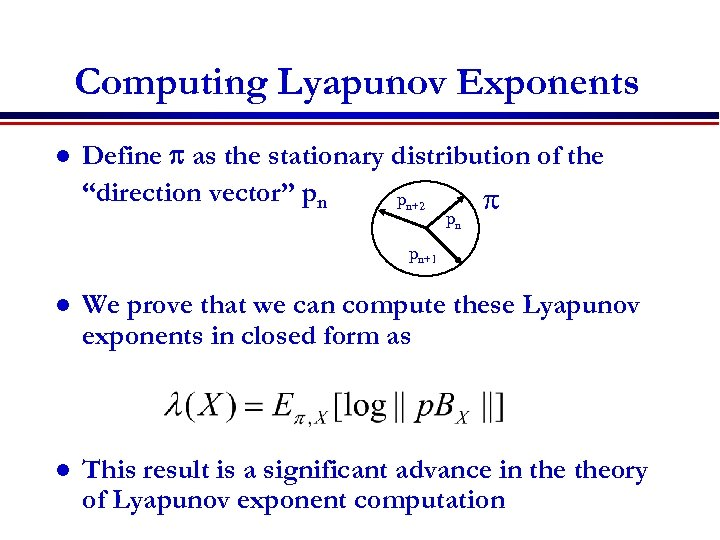Computing Lyapunov Exponents l Define p as the stationary distribution of the “direction vector” pnpn pn+2 p pn pn+1 l We prove that we can compute these Lyapunov exponents in closed form as l This result is a significant advance in theory of Lyapunov exponent computation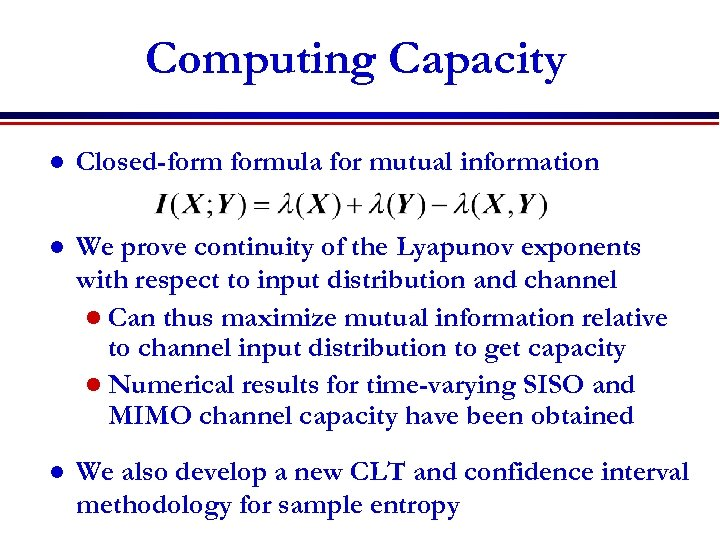Computing Capacity l Closed-formula for mutual information l We prove continuity of the Lyapunov exponents with respect to input distribution and channel l Can thus maximize mutual information relative to channel input distribution to get capacity l Numerical results for time-varying SISO and MIMO channel capacity have been obtained l We also develop a new CLT and confidence interval methodology for sample entropy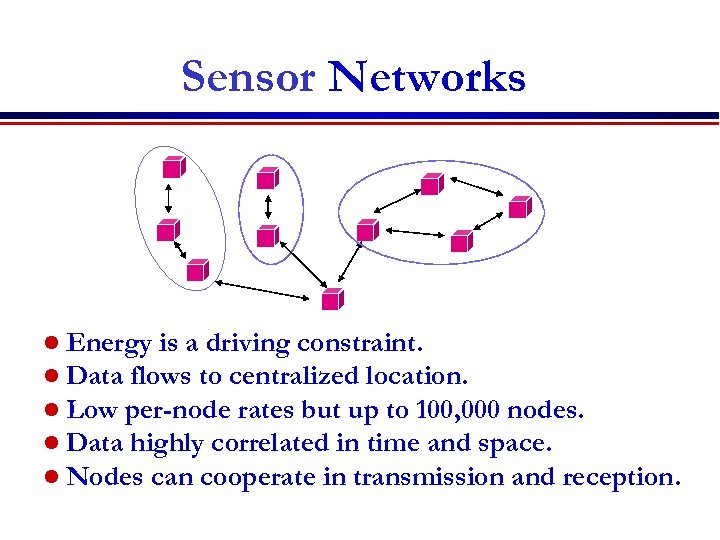Sensor Networks l Energy is a driving constraint. l Data flows to centralized location. l Low per-node rates but up to 100, 000 nodes. l Data highly correlated in time and space. l Nodes can cooperate in transmission and reception.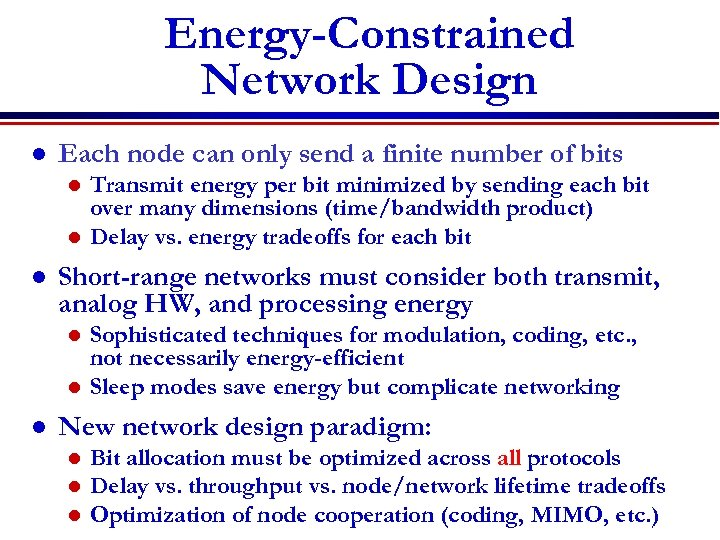Energy-Constrained Network Design l Each node can only send a finite number of bits Transmit energy per bit minimized by sending each bit over many dimensions (time/bandwidth product) l Delay vs. energy tradeoffs for each bit l l Short-range networks must consider both transmit, analog HW, and processing energy Sophisticated techniques for modulation, coding, etc. , not necessarily energy-efficient l Sleep modes save energy but complicate networking l l New network design paradigm: l l l Bit allocation must be optimized across all protocols Delay vs. throughput vs. node/network lifetime tradeoffs Optimization of node cooperation (coding, MIMO, etc. )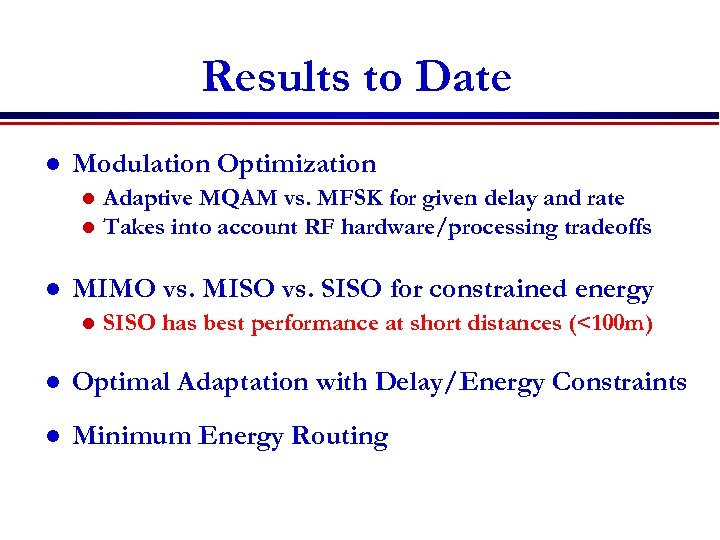Results to Date l Modulation Optimization l l l Adaptive MQAM vs. MFSK for given delay and rate Takes into account RF hardware/processing tradeoffs MIMO vs. MISO vs. SISO for constrained energy l SISO has best performance at short distances (<100 m) l Optimal Adaptation with Delay/Energy Constraints l Minimum Energy Routing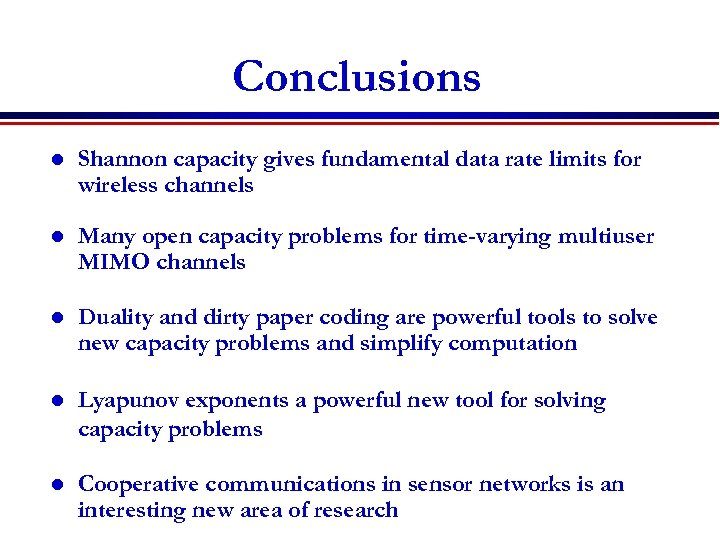Conclusions l Shannon capacity gives fundamental data rate limits for wireless channels l Many open capacity problems for time-varying multiuser MIMO channels l Duality and dirty paper coding are powerful tools to solve new capacity problems and simplify computation l Lyapunov exponents a powerful new tool for solving capacity problems l Cooperative communications in sensor networks is an interesting new area of research